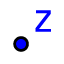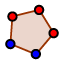# Construction of the Polygons

Yazar:
ilketopal
1. Construct a point and make a 4 sided regular polygon to represent digit value as 100.2. Construct 4 points and make a rectangle to represent digit value as 10. 3. Construct a point and make a square to represent digit value as 1. 4. The following script with a variable called "i" will give 10 vertical lines in bigger square. List (LineSegment (A+(0,i), A+(10,i)), i, 0, 10) 5. The following script with a variable called "i" will give 10 horizontal lines in bigger square. List (LineSegment (A+(i,0), A+(i,10)), i, 0, 10) 6. 9 polygons will be constructed by copying of already formed polygons and polygons' corners must be matched in the coordinate system.